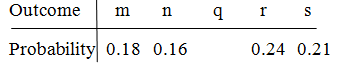# MA 121 Introduction to Statistics Coaching Session 2.1.1 # 15

1. Hi this is Asmita! I am a Student Success Coach and will be explaining some practice problems from the MA121 Introduction to Statistics course. Today we will review an assigned problem from Unit 2. In the “Homework Assessment” you were asked to solve this problem:

A sample space is S = {m, n, q, r, s}. Identify two events as U = {m, q, s} and V = {n, q, r}. The probabilities of some of the outcomes are given by the following table:Determine what P(q) must be.
Find P(U).
Find P(V).

a.
To find the probability in part a, use the following approach.

Write the given things.
Use the concept of sum of probabilities of sample space.
Plug the given individual probabilities.

Step 1: Write the given things:
S = {m, n, q, r, s}, P(m) = 0.18, P(n) = 0.16, P( r) = 0.24 and P(s) = 0.21

Step 2: Use the concept of sum of probabilities of sample space:
The sum of probabilities of outcomes of sample space must add up to 1.
That is, P(S) = 1

P(m) + P(n) + P(q) + P( r) + P(s) = 1

Step 3: Plug the given individual probabilities:
0.18 + 0.16 + P(q) + 0.24 + 0.21 = 1
0.79 + P(q) = 1
P(q ) = 1 - 0.79
P(q) = 0.21

Therefore, P(q) must be 0.21.

To find the probability of an event in part b and part c, use the following approach:
Write the given event and probabilities.
Use the concept of probability of an event to find the probability.
Plug the given individual probabilities, and find required probability.

b.
Step 1: Write the given event and probabilities:
U = {m, q, s}, P(m) = 0.18, P(q) = 0.21 and P(s) = 0.21

Step 2: Use the concept of probability of an event to find the probability:
The probability of an event is the sum of probabilities of the individual outcomes of that event.
That is, P(U) = P(m) + P(q) + P(s)

Step 3: Plug the given individual probabilities, and find P(U).
P(U) = 0.18 + 0.21 + 0.21
P(U) = 0.6
Therefore, P(U) = 0.6

c.
Step 1: Write the given event and probabilities:
V = {n, q, r}, P(n) = 0.16, P(q) = 0.21 and P( r) = 0.24

Step 2: Use the concept of probability of an event to find the probability:
The probability of an event is the sum of probabilities of the individual outcomes of that event.
That is, P(V) = P(n) + P(q) + P( r)

Step 3: Plug the given individual probabilities, and find P(V):

P(V) = 0.16 + 0.21 + 0.24
P(V) = 0.61
Therefore, P(V) = 0.61

Conclusion:
P(q) must be 0.21.
P(U) = 0.6
P(V) = 0.61

In addition to this, I am also attaching the pdf document of the practice problem for your ready reference.
Question 15.pdf (308.7 KB)

Please let me know if you have any question on this problem, or on this topic generally. I will be here in the forum for the next hour.